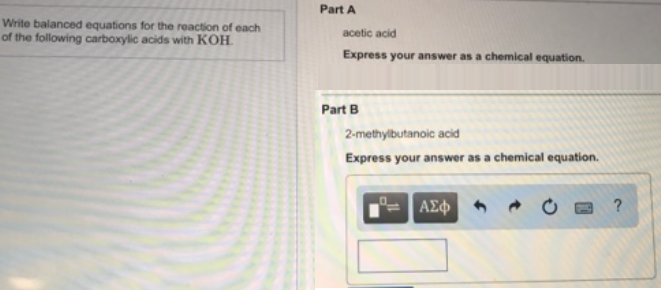# Problem: Write balanced equations for the reaction of each of the following carboxylic acids with KOH. Part A acetic acid Express your answer as a chemical equation. Part B 2-methylbutanoic acid Express your answer as a chemical equation.

###### FREE Expert Solution
83% (378 ratings)###### Problem Details

Write balanced equations for the reaction of each of the following carboxylic acids with KOH.

Part A

acetic acid

Express your answer as a chemical equation.

Part B

2-methylbutanoic acid

Express your answer as a chemical equation.Frequently Asked Questions

What scientific concept do you need to know in order to solve this problem?

Our tutors have indicated that to solve this problem you will need to apply the Acids and Bases concept. You can view video lessons to learn Acids and Bases. Or if you need more Acids and Bases practice, you can also practice Acids and Bases practice problems.

What professor is this problem relevant for?

Based on our data, we think this problem is relevant for Professor Urhoghide's class at MDC.